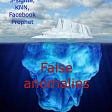# A Theoretical Perspective on XGBoost

Gradient Boosted Decision Tree (GBDT) is an ensemble-based method where trees are trained in sequence over residuals of the loss function. The main cost of GBDT is the construction of decision trees and the most time-consuming part is finding the best split points for each node. XGBoost¹, introduced in 2016, was an efficient implementation solving these challenges, and below is a look into the way it solved these issues.

The loss function is central to any supervised machine learning model and XGBoost is no exception. From the addition of new trees to splitting individual nodes in a tree, the loss function is the factor driving it all. Since each new tree is trying to minimize residual errors, the algorithm is similar across them.

## From Loss Function to Decision Tree

Loss Function and optimal leaf value: Consider a general tree structure in which we have T leaf nodes and each node as weight/value w. By taking a second-order approximation of the loss function we can rewrite it in the following form. The last two terms are regularization term which penalizes the number of leaf and value of leaf respectively.

f(x), g, and h are tree value, gradient, and hessian for each sample i in the dataset. Each of these samples will end up in one of T leaf nodes leaf weight will be predicted for that sample. Using this, we can rewrite the loss function in the following form.

In this form, the loss function becomes the sum of quadratic functions and it can attain a minimum value when each element of the sum i.e. leaf attains its minimum value.

The value of loss function (L*)at a minimum can be used as a score to judge tree structure and decision pruning tree or choosing a threshold for split can be made based on this measure.

Split Finding Algorithm: Each tree starts as a root node and then each node is split up into two nodes by selecting a feature and split point. XGBoost uses a pre-sorted algorithm, which sorts the feature and lists out candidate points by calculating the weighted percentile of that feature, where Hessians are used as weight. Error for each sample is weighted by hessian for that sample and can be seen by rewriting loss function in the following form :

Each node can then run a greedy search over the feature set and its candidate split points to select one with the maximum gain in tree score.

## Factors driving Speed

Both algorithmic choices and system design play a crucial part in speed up. One factor is the use pre-sorted algorithm mentioned above. Others are as mentioned below.

Handling sparse data: It's common to end up with a sparse dataset either because of one-hot encoding or due to missing values. Only non-missing values are considered for the split finding algorithm which reduces the computation complexity. For missing values, it can either go to left or right. Both cases are evaluated and the one with the best tree score is selected.

Column Block for Parallel Learning: For finding the best split on a feature, sorting is required and is the most time-consuming aspect. Each feature is sorted and stored in Compressed Column (CSC) format in in-memory units or blocks. Since the split-finding algorithm works on sorted data, rows can be divided into multiple blocks. Each block can be either on a different machine (for distributed learning) or stored on a disk when the data size is too large to fit in memory. Using this sorted structure, the quantile finding step becomes a linear scan over the sorted columns. This is valuable for local proposals at each node where candidates are generated frequently.

Cache-aware Access: Block structure helps in optimizing computation complexity of split finding but introduces another problem. Each sorted feature is now in a different order than the original data index. Gradient statistics for them are accessed in this non-continuous order which introduces overhead in time. To resolve this in the approximate algorithm, the optimum size of ²¹⁶ examples in each block is selected. Larger block size will result in cache miss as gradient statistics will not fit into the CPU cache.

## Comparison with LightGBM

LightGBM² was introduced by Microsoft to alleviate the unsatisfactory result of existing algorithms such as XGBoost for an even larger feature dimension. XGBoost needs to scan all data instances to check information gain for all features, it can be a bottleneck. Two novel technique was introduced to alleviate this:

Gradient-based One-Sided Sampling (GOSS): This concept is used to downsample the number of data instances on which to train any new decision tree. Gradient for any sample is a useful indicator of its importance. Low gradient means training error for that sample is small and it is already well trained. In the algorithm, this is used by only using top samples with the highest gradient and a random subset of low gradient samples. Low gradient samples still need to be included as it will change data distribution otherwise. Although information gain from low gradient samples is reduced by a factor to keep its importance low.

Exclusive Feature Bundling (EFB): In high dimensional data, a lot of features can be sparse and mutually exclusive (e.g. one-hot encoding). This exclusivity can be used to bundle them together and achieve speedup by reducing feature dimensions, without hurting performance.

1. Chen, T., & Guestrin, C. (2016, August). Xgboost: A scalable tree boosting system. In Proceedings of the 22nd acm sigkdd international conference on knowledge discovery and data mining (pp. 785–794).
2. Ke, G., Meng, Q., Finley, T., Wang, T., Chen, W., Ma, W., … & Liu, T. Y. (2017). Lightgbm: A highly efficient gradient boosting decision tree. Advances in neural information processing systems, 30, 3146–3154.

Let me know if you have questions or feedback in the comments.

--

--

--

## What is a Neural Network?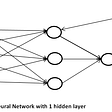## Run an inference with tflite-runtime inside a Raspberry Pi 4B.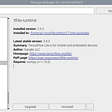## Semantic Search with S-BERT is all you need## Hinge Loss : [Machine Learning Bite Size Series]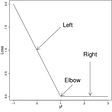## Calculating Number of Parameters in a LSTM Unit & Layer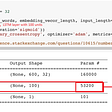## An Introduction to Advantage Actor-Critic method (A2C)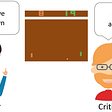## Fortifying the use of XGBoost with GPU## Understanding T5 Model : Text to Text Transfer Transformer Model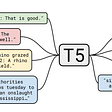## Know your Machine Learning Models Better With Model Interpretability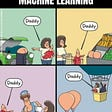## How is Time Series Forecasting different from Machine Learning?## How to Detect Anomalies — state-of-the-art methods using Conformal Prediction